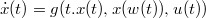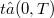﻿

### Metodo della deparametrizzazione applicato a problemi di controllo tipo ritardato

#### Abstract

We obtain an existence theorem for the minimum of the functional(Error rendering LaTeX formula) associated to the differential equation$\dot{x}(t)=g(t.x(t),x(w(t)),u(t))$ a.e.$t∈ (0,T)$, reducing our problem a simpler model where control variables are not present.

DOI Code: 10.1285/i15900932v2n2p235

Full Text: PDF